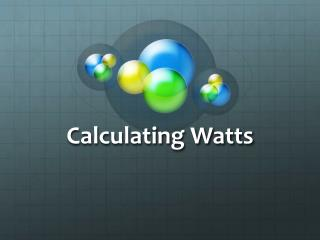# Calculating Watts - PowerPoint PPT PresentationDownload PresentationCalculating Watts

Calculating WattsDownload Presentation## Calculating Watts

- - - - - - - - - - - - - - - - - - - - - - - - - - - E N D - - - - - - - - - - - - - - - - - - - - - - - - - - -
##### Presentation Transcript

1. Calculating Watts

2. Watt Watt Watt?!?! • A watt is the basic unit of power. • Power is the rate at which electrical energy is delivered to a circuit. • “How many watts?” is a question often asked about speakers, sound systems, hair dryers, and light bulbs.

3. OHMMMMM…. • Ohms law states that voltage is the product of current and resistance. If you know two of the values, you can calculate the third. • The power formula is related to Ohms law. Use the power formula to calculate the current flow when a lamp is on. • The power formula is stated as: • P=E(I) • Where, • P=Power in Watts (W) • E=Operating Voltage • I=Current in amperes

4. What is the power for a light bulb with a voltage of 12.6 V and a current of 1.04 A? P=E(I) P=12.6V(1.04A)=13.1W Round the wattage to tenths, voltage to tenths, and current to hundredths. Rewrite the power formula in terms of the current. Solve for I. Start with P=E(I) Divide both sides by E. P/E=E(I)/E I=P/E Calculate the current in a bulb where the voltage is 14.5V and the power is 4.9W: I=P/E (4.9W)/(14.5V)=0.34A

5. A 1154 bulb has a double filament. The operating voltage is 6.4 V and the currents are 2.63 A and 0.75 A? What are the power values for each filament? For the lower-current filament: P=E(I)=6.4V(0.75A)=4.8W For the higher-current filament: P=E(I)=6.4V(2.63A)=16.8W

6. Don’t Get Frustrated!